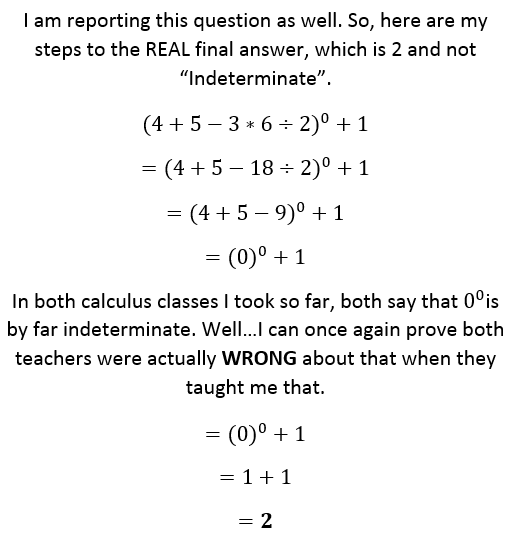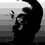# 0 to the 0th Power...In response to Juliet Hanson's problem.Note by Lew Sterling Jr
6 years, 3 months ago

This discussion board is a place to discuss our Daily Challenges and the math and science related to those challenges. Explanations are more than just a solution — they should explain the steps and thinking strategies that you used to obtain the solution. Comments should further the discussion of math and science.

When posting on Brilliant:

• Use the emojis to react to an explanation, whether you're congratulating a job well done , or just really confused .
• Ask specific questions about the challenge or the steps in somebody's explanation. Well-posed questions can add a lot to the discussion, but posting "I don't understand!" doesn't help anyone.
• Try to contribute something new to the discussion, whether it is an extension, generalization or other idea related to the challenge.

MarkdownAppears as
*italics* or _italics_ italics
**bold** or __bold__ bold
- bulleted- list
• bulleted
• list
1. numbered2. list
1. numbered
2. list
Note: you must add a full line of space before and after lists for them to show up correctly
paragraph 1paragraph 2

paragraph 1

paragraph 2

[example link](https://brilliant.org)example link
> This is a quote
This is a quote
    # I indented these lines
# 4 spaces, and now they show
# up as a code block.

print "hello world"
# I indented these lines
# 4 spaces, and now they show
# up as a code block.

print "hello world"
MathAppears as
Remember to wrap math in $$ ... $$ or $ ... $ to ensure proper formatting.
2 \times 3 $2 \times 3$
2^{34} $2^{34}$
a_{i-1} $a_{i-1}$
\frac{2}{3} $\frac{2}{3}$
\sqrt{2} $\sqrt{2}$
\sum_{i=1}^3 $\sum_{i=1}^3$
\sin \theta $\sin \theta$
\boxed{123} $\boxed{123}$

Sort by:

I read your response.though my English language is not very well, but I could get the answer of the question that was in my mind for a year. Thanks

- 6 years, 3 months ago

Welcome, Juliet. :)

- 6 years, 3 months ago

$0^0$ is indeterminate.

Depending on the scenario, sometimes people make the simplifying assumption that $0 ^ 0 = 0$ or 1, to help make their calculations work in special cases.

Staff - 6 years, 3 months ago

Graph it for a second and see what it says.

- 6 years, 3 months ago

Graphing it, however, still begs the question of whether 0^0 is indeterminate or not. If it's indeterminate, then there is no point at x=0. The graph of ((x^2)-1)/(x-1), for example, has a hole at x=1, even though its limit as x approaches 1 equals 2.

- 6 years, 3 months ago

Could you please give a special case in which the simplifying assumption that 0^0 = 0 helped make a calculation work?

- 6 years, 3 months ago

Incorrect. The zero power axiom trumps the zero multiplicity rule.

- 5 years, 5 months ago

how the hell can 0^0 be indeterminate anyway? You take nothing and multiply it by nothing and should give you nothing. I always thought that any number ^0 = 0...

- 6 years, 3 months ago

@Ricky Tang , normally, any number to the 0th power is 1. Type 0^0 in both the Google calculator and Desmos.com/calculator (Hint: also graph x^x on desmos) and let me know what both say.

- 6 years, 3 months ago

I know what everyone is saying is true but it just doesn't make any sense. Anything times nothing should be nothing, considering any number multiplied by 0 is 0 so why isnt it the same for a number compound to 0? I love math but this rule simply doesn't make any sense.

- 6 years, 3 months ago

I have a question for you: Isn't nothing considered a "something"?

- 6 years, 3 months ago

That's because exponentiation is not just compounding; it could also be discounting (or whatever you call the opposite of compounding). When you raise a number to a power greater than $1$, you compound that number. It's like asking, "If I compound what I have now, how much will I have then?" On the other hand, when you raise a number to a power less than $1$, you discount that number. It's like asking, "If what I have now is a result of compounding, then how much have I had before?"

- 5 years, 8 months ago

Multiplication is just repetitive addition of the same number, which is why we evaluate it first before we do addition.

Let x be any real number.

3(x) = x + x + x

2(x) = x + x

1(x) = x

0 is the additive identity number of the set of real numbers, which means that it is always there in the world of addition, though we just take it for granted.

3(x) = 0 + x + x + x

2(x) = 0 + x + x

1(x) = 0 + x

You can add as many zeros you want, and it would be like you've just pumped in some air for the x's to breathe.

3(x) = 0 + 0 + x + x + x

2(x) = 0 + 0 + x + x

1(x) = 0 + 0 + x

And what happens when we don't add x to a world of zeros?

0(x) = 0 + 0

Exponentiation, on the other hand, is just repetitive multiplication of the same number, which is why we evaluate it first before we do multiplication.

x^3 = (x)(x)(x)

x^2 = (x)(x)

x^1 = x

1 is the multiplicative identity number of the set of real numbers, which means that it is always there in the world of multiplication, though we just take it for granted. Ever wondered why prime number is defined to be greater than 1?

x^3 = 1(x)(x)(x)

x^2 = 1(x)(x)

x^1 = 1(x)

You can multiply by as many ones you want, and it would be like you've just pumped in some air for the x's to breathe.

x^3 = (1)(1)(x)(x)(x)

x^2 = (1)(1)(x)(x)

x^1 = (1)(1)(x)

And what happens when we don't multiply a world of ones by x?

x^0 = (1)(1)

- 6 years, 3 months ago

Nothing multiplied by nothing is nothing, which is a something. :)

- 5 years, 8 months ago

I would just like to state something that my teachers have taught me over the years. They always tell all of their students to look at exponents as a pattern, for example: 2^5 = 32 2^4 = 16 2^3 = 8 2^2 = 4 2^1 = 2 2^0 = 1 2^-1 = 1/2

... and so on and so forth. The way to get each descending term there is to divide by two to get your next term, which should make sense because you multiply the previous term each time to get the next ascending term. With this pattern in mind, 2^0 has to equal 1, and it works the same with any other number raised to the power of 0. Because of this there is no doubt in my mind that x^0 = 1.

- 6 years, 3 months ago

Yes i agree

- 6 years, 3 months ago

They weren't wrong in teaching you that, calculus is a stepping stone into understanding mathematics that are/were developmental. It's a gradual process. 0^0 was indeterminate until other rules from different countries and mathematicians created new formulas, so you have to change from one school of thought to another to arrive at a solution. Math despite our best attempts isn't always uniform, it depends on who's method you are studying and why. Just my two cents.

- 5 years, 7 months ago

wait does anyone realize that 0^x=0 and x^0=1 so original poster is meh

- 1 year, 8 months ago

hech no! when x approaches 2. x² = 10000. So. 5 = 23 and b = 3. it's actually pretty easy to get the limit as x approaches 3. But. an equation can get pretty tough. especially if it has x² in it. so. how can we change that? we do this: we subtract by x. Then. we use (1 + 1)² (2 + 2)². Last but not least. we add 37.

- 1 month, 3 weeks ago